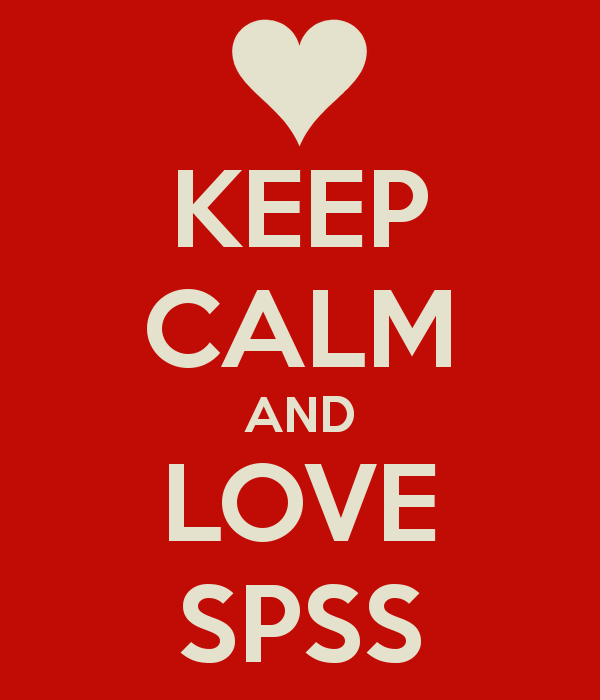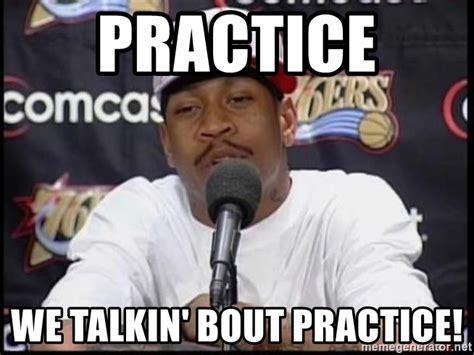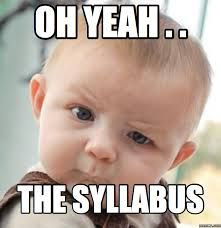# Practice and Assignments## Assignment: t-test for Dependent/Paired Samples (SPSS)

In this assignment (created by Dr. Kimberly A. Barchard and Dr. Leiszle Lapping-Carr), students learn how to use SPSS to compare dependent groups using the dependent samples t-test and using confidence intervals for the difference of the means. A detailed grading rubric is included. This assignment was designed to be completed with an existing dataset …## Assignment: Single Sample t-test (SPSS)

In this assignment (created by Dr. Kimberly A. Barchard and Dr. Leiszle Lapping-Carr), students learn how to use SPSS to conduct a single sample t-test and calculate a confidence interval and how to interpret the output for both. A detailed grading rubric is included. This assignment was designed to be completed with an existing dataset …## Practice: Measuring Dependent Variables: Types and Scales of Measurement

This is a brief in-class practice activity (created by Raechel Soicher) to help students review different types of measurement for dependent variables as well as identify scales of measurement. Raechel recommends teaching about the different ways to measure a dependent variable before giving students the opportunity to review using this brief worksheet. She provides definitions …## Practice: Having Fun with Operational Definitions

One of the challenges instructors face when discussing conceptual and operational definitions of variables is helping students to understand that the operational definition of a variable involves measuring the variable in a concrete, quantitative manner. The practice activity asks students to identify the relevant variables of interest and then suggest ways that these variables can …

## Practice: Calculating the Pearson r Correlation (Video Games and GPA)

Instructors should assign this problem (about how many hours per week they played video games and their GPA) to students as in-class practice or homework after students have learned how to calculate a Pearson r correlation and test for significance. The activity leads students through determining the hypotheses, calculating the correlation coefficient, making a decision …

## Practice: Calculating the Pearson r Correlation (Math and Spelling Ability)

Instructors should assign this problem (about scores on a math and a spelling test) to students as in-class practice or homework after students have learned how to calculate a Pearson r correlation and test for significance. The activity leads students through determining the hypotheses, calculating the correlation coefficient, making a decision about the null hypotheses …

## Practice: Calculating the Pearson r Correlation (Height and Running Speed)

Instructors should assign this problem (about how height and running speed in the 40 yard dash) to students as in-class practice or homework after students have learned how to calculate a Pearson r correlation and test for significance. The activity leads students through determining the hypotheses, calculating the correlation coefficient, making a decision about the …

## Practice: Calculating the t-test for Independent Samples/Means (Temperature and Mood)

Instructors should assign this problem (about how temperature impacts mood) to students as in-class practice or homework after students have learned how to calculate a t-test for independent means and test for significance. The activity leads students through determining the type of statistical analysis to use, the hypotheses, calculating the t-ratio and effect size (if …

## Practice: Calculating the t-test for Independent Samples/Means (Research Methods and GPA)

Instructors should assign this problem (about how taking research methods impacts GPA) to students as in-class practice or homework after students have learned how to calculate a t-test for independent means and test for significance. The activity leads students through determining the type of statistical analysis to use, the hypotheses, calculating the t-ratio and effect …

## Practice: Calculating the t-test for Paired-Samples/Dependent Means (Drugs and Learning)

Instructors should assign this problem (about drugs and learning) to students as in-class practice or homework after students have learned how to calculate a t-test for dependent means and test for significance. The activity leads students through determining the type of statistical analysis to use, the hypotheses, calculating the t-ratio and effect size (if appropriate), …

## Lecture Slides: Measuring Dependent Variables

This  lecture introduces students to several different ways of measuring   behavior as the dependent variable.  Several types of behavioral measures are shown, including: behavioral trace, behavioral observation, and behavioral choice. The goal of this lecture is to get students to see other types of measuring DVs, aside from self-report. This starts with a quick overview …

## Lecture Slides: Manipulating Independent Variables

This  lecture introduces students to several different ways of manipulating independent variables. Some techniques covered include: mock Facebook profiles, priming, having participants count money, and viewing cute pictures. The goal of this lecture is to get students to see a variety of ways that researchers can manipulate IVs. Each example provides the citation for the …

## Lecture Slides: Calculating the Pearson r Correlation (Study Hours and GPA)

The goal of this PowerPoint is to work through the step-by-step process of hand calculating a Person Correlation. The slides start with the formula involved and then a sample problem is presented. It is designed for you to have the class solve the problem along with you as you advance through the slides and animation. …

## Lecture Slides: Calculating the t-test for Paired-Samples/Dependent Means (Weight Loss Study)

The goal of this PowerPoint is to work through the step-by-step process of hand calculating a t-test for dependent means. The slides start with an explanation of the formula involved and then a sample problem is presented. It is designed for you to have the class solve the problem along with you as you advance …## Assignment: Creating a Conference Poster on Sex Differences (SPSS)

In this assignment (created by Dr. Kimberly A. Barchard and Dr. Leiszle Lapping-Carr), students conduct a literature review, analyze data using an independent samples t-test and a confidence interval, create a grouped frequency distribution and a histogram, write a research report, and create a PowerPoint poster. A detailed grading rubric is included. This assignment was …## Example Syllabus – Statistics with Lab

A sample syllabus for an introductory statistics course. Though this course has a lab, that content could also go into the lecture portion of the course, or incorporated as something students do outside of class as group projects. The syllabus also describes a statistical presentation assignment, as well as a Statistics Portfolio where students curate …

## Practice: Calculating the t-test for Paired-Samples/Dependent Means (Thinking Logically Over Time)

Instructors should assign this problem (about graduate programs training students to think logically) to students as in-class practice or homework after students have learned how to calculate a t-test for dependent means and test for significance. The activity leads students through determining the type of statistical analysis to use, the hypotheses, calculating the t-ratio and …